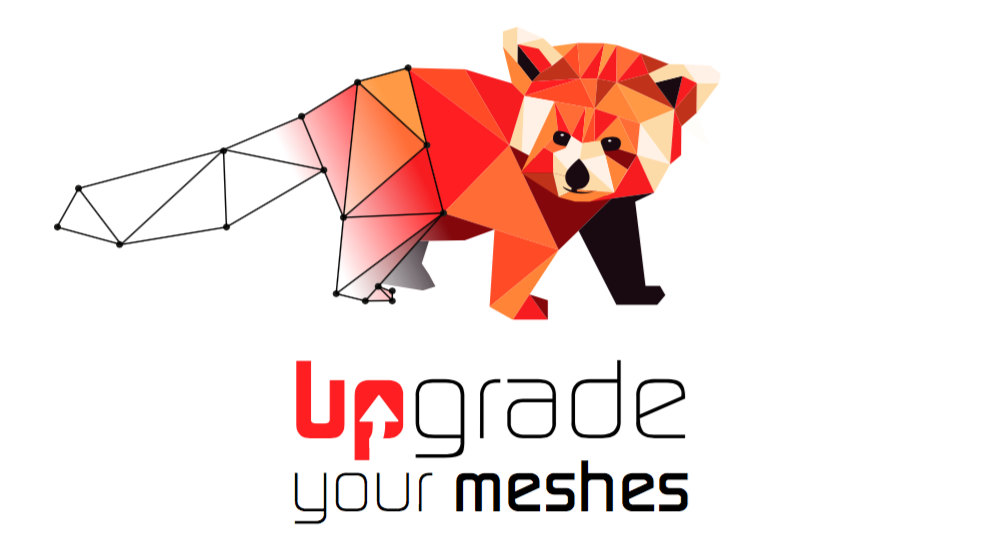# Meaning of "mesh quality" in anisotropic output result

Hi to all,

probably it is a trivial question, but I don’t understand how to interpret the “mesh quality” section in the output results after an anisotropic mesh adaptation.

For example:

BEST 0.999487
AVERAGE 0.723562
WORST 0.142121

95,94% > 0.5

What does it mean “best=0.999487” ?

Thank you for any help!

Hi,

You will find a description of Mmg histograms on the Mmg webpage : Mmg histograms – Mmg Platform

Do not hesitate if you still have questions.

Best Regards,
Algiane

yes i read this part, but it does not explain in which sense “best” is intended. How it is calculated? What is its relation with , for example, the volume of the tetrahedra or the stretching factors?

Can you explain me in more detail the idea behind this interesting output result?

Thanks

Ok, I will try to add some formula and explanation on the webpage.

So, the quality in Mmg is a measure of the shape ratio in the metric space reconstructed from the size map.

A “perfect” element has quality 1. Quality 0 correspond to element with volume null.
For an isotropic metric an element of quality 1 is equilateral, for an anisotropic metric it is inscribed in the metric tensor.

More precisely, if I note e_i the i^{th} edges of an element, l_i their lengths, \bar{M} the mean metric over this element, V_{\bar{M}_i} the volume of this element in the mean metric:

• quality formula for a triangle is:
Q_T = \alpha \frac{V_{\bar{M}}}{\sum_{e_i} l_i^2}
• quality formula for a tetra is:
Q_T = \alpha \frac{V_{\bar{M}}}{(\sqrt{\sum_{e_i} l_i^2})^{\frac{3}{2}}}

with \alpha a normalization coefficient such as quality is 1 for an isotropic metric and and equilateral element.

Hope that it helps,

Best Regards,
Algiane

ok now is much more clear. In other words, we can say that the quality quantify how well the metric is reproduced in the mesh, correct?

Hi,

Yes, we can say that, while keeping in mind that it translates the deformation of the element (in the metric).

Best

1 Like